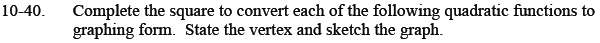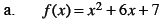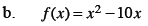Home > CCA2 > Chapter 10 > Lesson 10.1.2 > Problem10-40

10-40.
1. Complete the square to convert each of the following quadratic functions to graphing form. State the vertex and sketch the graph. Homework Help ✎

1. f(x) = x2 + 6x + 7

2. f(x) = x2 − 10xMove the constant to the other side.

Determine what number should be added to both sides so that the right side is factorable as (xh)2.

Add 9 to both sides.

Factor the right side of the equation.

Move the '2' back to the right side.

Vertex: (−3, −2) Why/how do you know?

f(x) − 7 = x2 + 6x

f(x) + 2 = x2 + 6x + 9

f(x) + 2 = (x + 3)2

f(x) = (x + 3)2 − 2Follow the steps outlined in part (a).